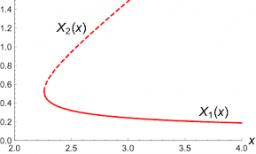# Calculate 4795

Calculate the larger of the two addends if you know that the smaller census is a third of the larger one, and their sum is 48

b =  36

### Step-by-step explanation:

a+b = 48
a = b/3

a+b = 48
3a-b = 0

Pivot: Row 1 ↔ Row 2
3a-b = 0
a+b = 48

Row 2 - 1/3 · Row 1 → Row 2
3a-b = 0
1.33b = 48

b = 48/1.33333333 = 36
a = 0+b/3 = 0+36/3 = 12

a = 12
b = 36

Our linear equations calculator calculates it.Did you find an error or inaccuracy? Feel free to write us. Thank you!

Tips for related online calculators
Do you have a system of equations and looking for calculator system of linear equations?